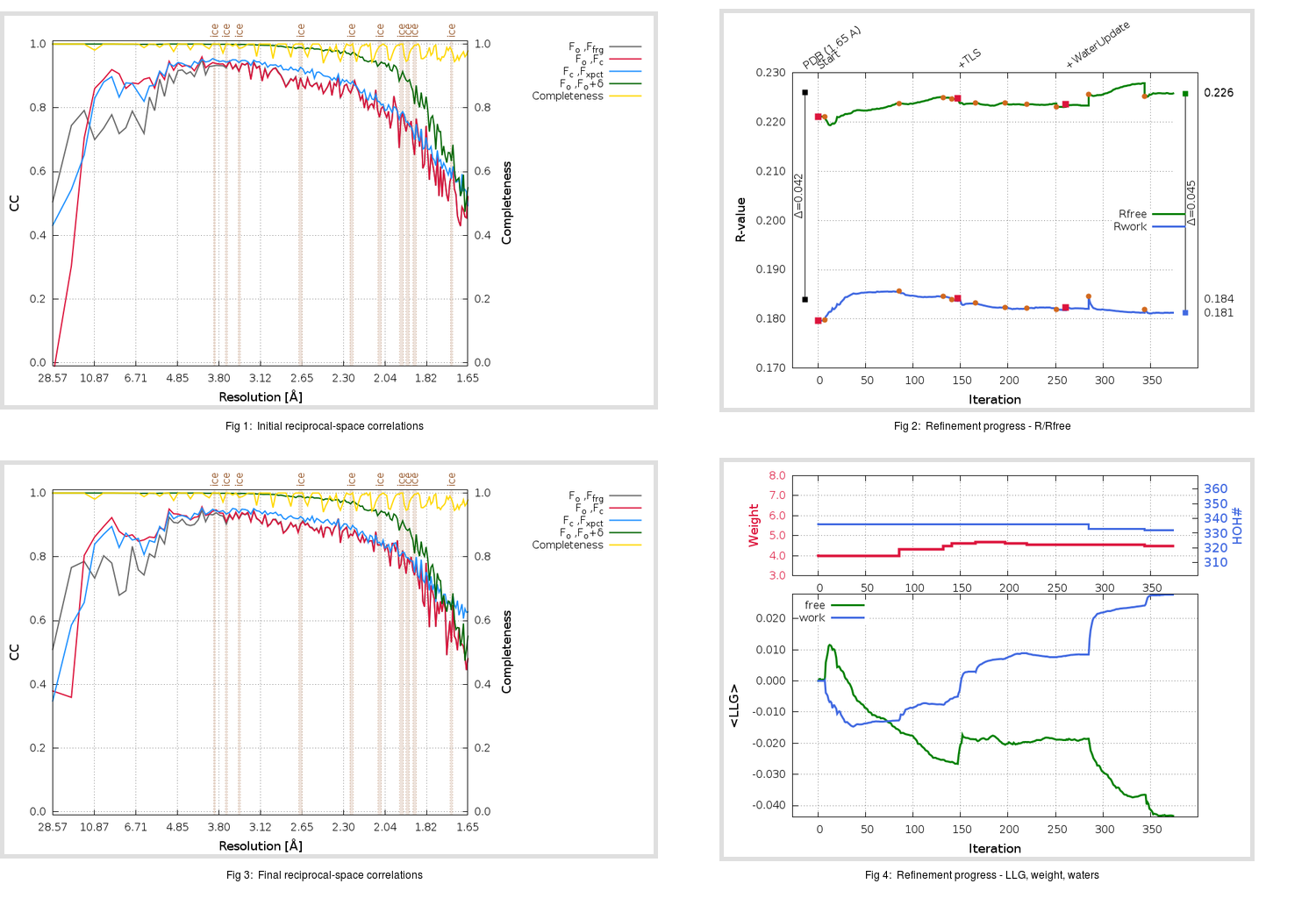Content:

```    Diffraction limits & principal axes of ellipsoid fitted to diffraction cut-off surface:
1.812         0.9937   0.0000  -0.1117       0.991 a* - 0.130 c*
1.733         0.0000   1.0000   0.0000       b*
1.555         0.1117   0.0000   0.9937       0.286 a* + 0.958 c*
```

## Deposited

` `
 Date deposited Date data collection Resolution R, Rfree 20200315 20200304 1.65 0.1810 0.2260

Molprobity (CCP4 7.0 version) summary:

```Ramachandran outliers =   0.33 %
favored =  98.34 %
Rotamer outliers      =   0.38 %
C-beta deviations     =     0
Clashscore            =   5.07
RMS(bonds)            =   0.0132
RMS(angles)           =   1.82
MolProbity score      =   1.27
Resolution            =   1.65
R-work                =   0.1810
R-free                =   0.2260
```

```Number of waters      =   336

<B> (all atoms) =   24.02 ( sd =    9.18 ) for       2737 non-hydrogen atoms
<B>   (protein) =   22.48 ( sd =    8.27 ) for       2370 non-hydrogen atoms
<B>     (water) =   33.78 ( sd =    9.21 ) for        336 non-hydrogen atoms
<B>    (others) =   36.35 ( sd =    8.16 ) for         31 non-hydrogen atoms

B min/max       (all non-hydrogen atoms) =   12.44 /   68.62
B min/max   (protein non-hydrogen atoms) =   12.56 /   68.62
B min/max     (water non-hydrogen atoms) =   12.44 /   61.04
B min/max     (other non-hydrogen atoms) =   24.93 /   49.72
```

## BUSTER (re-)refinement

` `

Molprobity (CCP4 7.0 version) summary:

```Ramachandran outliers =   0.33 %
favored =  98.34 %
Rotamer outliers      =   0.38 %
C-beta deviations     =     0
Clashscore            =   2.32
RMS(bonds)            =   0.0116
RMS(angles)           =   1.57
MolProbity score      =   1.01
Resolution            =   1.65
R-work                =   0.1812
R-free                =   0.2258
```

```Number of waters      =   332

<B> (all atoms) =   25.54 ( sd =    8.72 ) for       2733 non-hydrogen atoms
<B>   (protein) =   23.82 ( sd =    7.13 ) for       2370 non-hydrogen atoms
<B>     (water) =   36.97 ( sd =   10.14 ) for        332 non-hydrogen atoms
<B>    (others) =   34.66 ( sd =    8.67 ) for         31 non-hydrogen atoms

B min/max       (all non-hydrogen atoms) =   11.84 /   72.48
B min/max   (protein non-hydrogen atoms) =   11.84 /   65.13
B min/max     (water non-hydrogen atoms) =   14.12 /   72.48
B min/max     (other non-hydrogen atoms) =   26.48 /   42.84
```

Refinement progression:Results:

` `
 File Remark 5RES_aB_refine.01_03_refine.pdb.gz exact refinement commands are in header 5RES_aB_refine.01_03_refine.mtz.gz including original deposited data and several re-refinement map coefficients 5RES_aB_refine.01_03_BUSTER_model.cif.gz including any non-standard compound restraints 5RES_aB_refine.01_03_BUSTER_refln.cif.gz